NEET  >  Test: Electrostatic Potential

# Test: Electrostatic Potential

Test Description

## 20 Questions MCQ Test Physics Class 12 | Test: Electrostatic Potential

Test: Electrostatic Potential for NEET 2022 is part of Physics Class 12 preparation. The Test: Electrostatic Potential questions and answers have been prepared according to the NEET exam syllabus.The Test: Electrostatic Potential MCQs are made for NEET 2022 Exam. Find important definitions, questions, notes, meanings, examples, exercises, MCQs and online tests for Test: Electrostatic Potential below.
Solutions of Test: Electrostatic Potential questions in English are available as part of our Physics Class 12 for NEET & Test: Electrostatic Potential solutions in Hindi for Physics Class 12 course. Download more important topics, notes, lectures and mock test series for NEET Exam by signing up for free. Attempt Test: Electrostatic Potential | 20 questions in 20 minutes | Mock test for NEET preparation | Free important questions MCQ to study Physics Class 12 for NEET Exam | Download free PDF with solutions
 1 Crore+ students have signed up on EduRev. Have you?
Test: Electrostatic Potential - Question 1

### The minimum velocity v with which charge q should be projected so that it manages to reach the centre of the ring starting from the position shown in figure is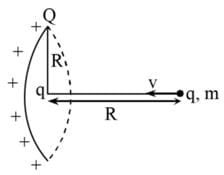Detailed Solution for Test: Electrostatic Potential - Question 1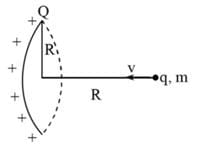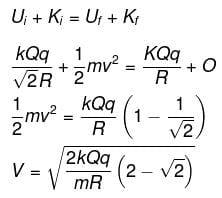Test: Electrostatic Potential - Question 2

### The work done in moving a positive test charge qo from infinity to a point P at a distance r from the charge q is

Detailed Solution for Test: Electrostatic Potential - Question 2

Definition based

Test: Electrostatic Potential - Question 3

### Electric field intensity is equal to

Detailed Solution for Test: Electrostatic Potential - Question 3

Definition based

Test: Electrostatic Potential - Question 4

Electric potential at a point located far away from the charge is taken to be

Detailed Solution for Test: Electrostatic Potential - Question 4

Electric potential at a point located far away from the charge is taken to be zero Because the distance is much larger so in other words there is no effect of other charges on that charge.

Test: Electrostatic Potential - Question 5

A charge of 6 mC is located at the origin. The work done in taking a small charge of -2 x 10-9 C from a point P (0, 3 cm, 0) to a Q (0,4 cm, 0) is​

Detailed Solution for Test: Electrostatic Potential - Question 5

q=6x10-10 c
Q=-2x10-9c
r1=3x10-2m
r2=4x10-2m
△vQ=w/q
(1/4πεo)-2x10-9/10-2[(1/4)-(1/3)]=W/6x10-3
9x109x2x10-9x6x10-3/12x10-2=W
W=9x10-3x102
W=0.9J

Test: Electrostatic Potential - Question 6

A particle of mass 1 Kg and charge 1/3 μC is projected towards a non conducting fixed spherical shell having the same charge uniformly distributed on its surface. The minimum intial velocity V0 of projection of particle required if the particle just grazes the shell is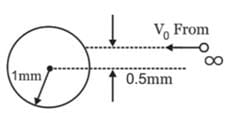Detailed Solution for Test: Electrostatic Potential - Question 6

From conservation of angular momentum,
mr0 = r/2 = mvr
⇒ v = v0/2
From conservation of energy,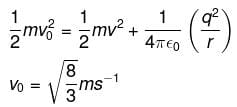Test: Electrostatic Potential - Question 7

On moving a charge of 20 coulombs by 2 cm, 2 J of work is done, then the potential difference between the points is

Detailed Solution for Test: Electrostatic Potential - Question 7

Potential difference between two points is given by

Va - Vb = W/q0

Work, W = 2 J

Charge, q0 = 20 C

Potential difference = 2/20 = 0.1 V

The correct option is C.

Test: Electrostatic Potential - Question 8

The amount of work done in moving a charge from one point to another along an equipotential line or surface charge is

Detailed Solution for Test: Electrostatic Potential - Question 8

Since Potential difference between two points in equipotential surfaces is zero, the work done between two points in equipotential surface is also zero.

Test: Electrostatic Potential - Question 9

A charge is uniformly distributed inside a spherical body of radius r1 = 2r0 having a concentric cavity of radius r2 = r0 (ρ is charge density inside the sphere). The potential of a point P or  a distance 3r0/2 from the centre is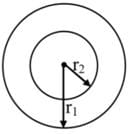Detailed Solution for Test: Electrostatic Potential - Question 9

Electric field at a distance r,
(r0 < r < 2r0) from the center is given by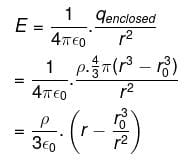Potential at the outer surface,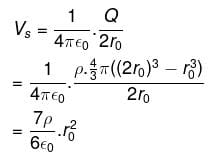∴ If V is the required potential at r  = 3r0/2,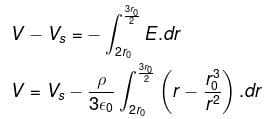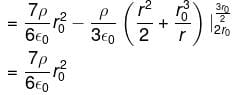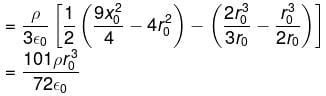Test: Electrostatic Potential - Question 10

Electric field intensity at point ‘B’ due to a point charge ‘Q’ kept at a point ‘A’ is 12 NC-1 and the electric potential at a point ‘B’ due to same charge is 6 JC-1. The distance between AB is​

Detailed Solution for Test: Electrostatic Potential - Question 10

E.l = V   where,
E = electric field intensity = 12 N/C
V = electric potential = 6 J/C
=> distance between A and B,
l = (6 / 12) m or (1 / 2) m = 0.5 m

Test: Electrostatic Potential - Question 11

E = -dV/dr, here the negative sign signifies that​

Detailed Solution for Test: Electrostatic Potential - Question 11

Solution :- The negative sign is just a convention and it signifies that the direction of E is opposite to the direction in which potential increases.

Test: Electrostatic Potential - Question 12

Work done in carrying 2C charge in a circular path of radius 2m around a charge of 10C is​

Detailed Solution for Test: Electrostatic Potential - Question 12

The overall work performed in carrying a 2coulomb charge in a circular orbit of radius 3 m around a charge of 10 coulomb is calculated below.
It is a well-known fact that W=qdv.
Here dV is the change in overall potential. In the circular orbit of r potential at each point is similar.
Most significantly, the value of r is 3.
The value of dv=0 and hence W=q0=0.

Test: Electrostatic Potential - Question 13

Dimensional formula for potential difference is

Detailed Solution for Test: Electrostatic Potential - Question 13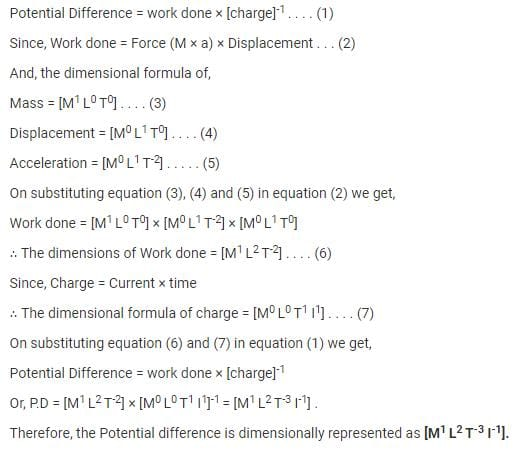Test: Electrostatic Potential - Question 14

A point charge 2nC is located at origin. What is the potential at (1,0,0)?

Detailed Solution for Test: Electrostatic Potential - Question 14

V = Q/(4πεr), where r = 1m
V = (2 X 10-9)/(4πε x 1) = 18 volts.

Test: Electrostatic Potential - Question 15

If 100 J of work has to be done in moving an electric charge of 4C from a place where potential is -5 V to another place, where potential is V volt. The value of V is

Detailed Solution for Test: Electrostatic Potential - Question 15

From the definition, the work done to a test charge ‘q0’ from one place to another place in an electric field is given by the formula
W=q0x[vfinal-vinitial ]
100=4x[v-(-5)]
v+5=25
v=20V

Test: Electrostatic Potential - Question 16

A particle of charge q1 = 3μC is located on x-axis at the point x1 = 6 cm. A second point charge q2 = 2μC is placed on the x-axis at x2 = -4 cm. The absolute electric potential at the origin is​

Detailed Solution for Test: Electrostatic Potential - Question 16

V=kq/r
Therefore, Vnet=Kx[(2µc/4) + (3µc/6)]
=9c109x10-6[1/2+1/2]x102
=9x105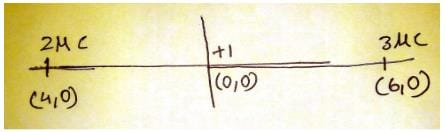Test: Electrostatic Potential - Question 17

Electric potential is

Detailed Solution for Test: Electrostatic Potential - Question 17

Electric potential is the electric potential energy per unit charge. In equation form, V=U/q, where U is the potential energy, q is the charge, and V is the electric potential. Since both the potential energy and charge are scalar quantities, so does the potential.
And it's dimension [ L2M1T-3I-1]

Test: Electrostatic Potential - Question 18

A long, hollow conducting cylinder is kept coaxially inside another long, hollow conducting cylinder of larger radius. Both the cylinders are initially electrically neutral.

Detailed Solution for Test: Electrostatic Potential - Question 18

When the charge is given to inner cylinder than there is an electric field is produced between cylinders which is given by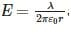and due to this a potential difference is developed between two cylinders.

Test: Electrostatic Potential - Question 19

Equal charges are given to two spheres of different radii. The potential will

Detailed Solution for Test: Electrostatic Potential - Question 19

When equal charges are given to two spheres of different radii, the potential will be more or the smaller sphere as per the equation, Potential = Charge / Radius.
Since potential is inversely proportional to radius, the smaller radius will have higher potential and vice versa.

Test: Electrostatic Potential - Question 20

Consider a solid cube made up of insulating material having a uniform volume charge density. Assuming the electrostatic potential to be zero at infinity, the ratio of the potential at a corner of the cube to that at the centre will be

Detailed Solution for Test: Electrostatic Potential - Question 20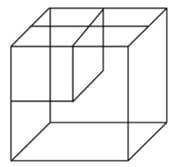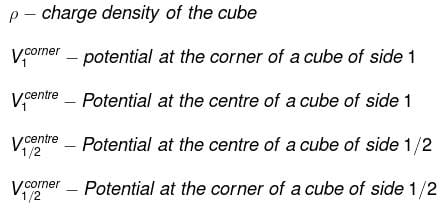By dimensional analysis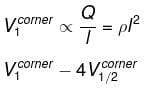but by superposition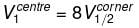Because of the centre of the larger cube lies at a corner of the eight smaller cubes of which it is made
therefore,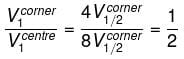## Physics Class 12

157 videos|425 docs|213 tests
 Use Code STAYHOME200 and get INR 200 additional OFF Use Coupon Code
Information about Test: Electrostatic Potential Page
In this test you can find the Exam questions for Test: Electrostatic Potential solved & explained in the simplest way possible. Besides giving Questions and answers for Test: Electrostatic Potential, EduRev gives you an ample number of Online tests for practice

## Physics Class 12

157 videos|425 docs|213 tests

### How to Prepare for NEET

Read our guide to prepare for NEET which is created by Toppers & the best Teachers